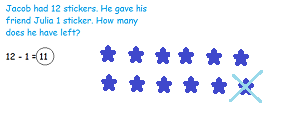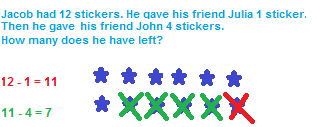# Two-Step Math Word Problems

An error occurred trying to load this video.

Try refreshing the page, or contact customer support.

Coming up next: How to Borrow in Math

### You're on a roll. Keep up the good work!

Replay
Your next lesson will play in 10 seconds
• 0:02 What Are Math Word Problems?
• 1:05 Solving Two-Step Math…
• 2:22 Practice Problem
• 3:33 Lesson Summary
Save Save

Want to watch this again later?

Timeline
Autoplay
Autoplay
Speed Speed

#### Recommended Lessons and Courses for You

Lesson Transcript
Instructor: Emily Hume

Emily is a Reading Specialist and Literacy coach in a public elementary school with a Master's Degree in Elementary Education.

You might be pretty comfortable solving math word problems with just one step, but have you tried a two-step problem? They're not as tough as you might think! This lesson will help you learn how to tackle those two-step word problems with ease!

## What Are Math Word Problems?

A math word problem is a math problem in which the information needed to solve the problem is provided using words rather than numbers or symbols. It requires you to read or listen before solving the problem using your math skills. Here's an example of a basic math word problem that may look familiar to you:Many math word problems only have one step, like this example that requires you to subtract 1 from 12. But be careful - sometimes word problems have two steps. Two-step math word problems require you to solve two equations (or number sentences) before you come to an answer. The problem might have two different operations (like multiplication and addition), or it might have two of the same operation, like this one that requires subtraction twice:## Solving Two-Step Math Word Problems

Begin your two-step word problem like you would any other word problem by reading it carefully to decide what you need to do, including which step to complete first. Re-read the problem if necessary. Then, work through the first step. Look at the important information in the problem, and turn that information into an equation by translating the words into operations. Feel free to draw pictures to help you through the problem.

Let's try this on our two-step example word problem:Jacob gave Julia 1 sticker. This translates to the equation 12 - 1, which equals 11. So, 11 is the answer to our first step.

Now, re-read the problem to make sure you're on the right track and then work through your second step. Again, try to turn the word problem into an equation. In the example problem, Jacob gives 4 of his 11 remaining stickers away to John. So, we can turn this word problem into the equation 11 - 4, which equals 7. So, 7 is the answer to our second step and the word problem.

After completing the problem, make sure to check your work and reread the question in the word problem and ask yourself, 'Did I answer the question?'

To unlock this lesson you must be a Study.com Member.

### Register to view this lesson

Are you a student or a teacher?

#### See for yourself why 30 million people use Study.com

##### Become a Study.com member and start learning now.
Back
What teachers are saying about Study.com

### Earning College Credit

Did you know… We have over 200 college courses that prepare you to earn credit by exam that is accepted by over 1,500 colleges and universities. You can test out of the first two years of college and save thousands off your degree. Anyone can earn credit-by-exam regardless of age or education level.